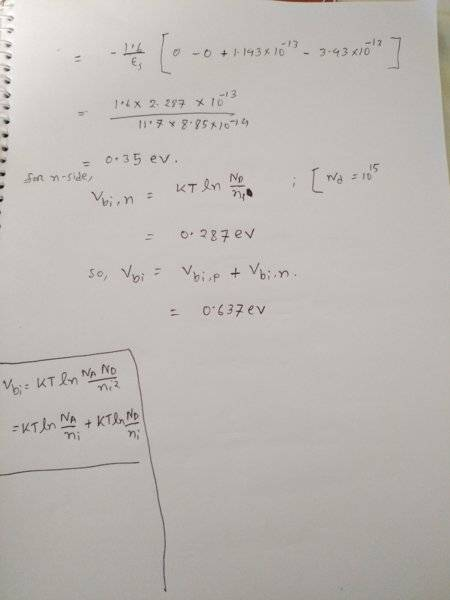# Non uniformly distributed p-n junction related problem

• THE HARLEQUIN
In summary, a diffused silicon p-n junction has a linearly graded junction on the p side with a = 2 x10^19 cm-4, and a uniform doping of 10^15 cm-3 on the n side. If the depletion width on the p side is 0.7 micro meter at zero bias, ﬁnd the total depletion width, built-in potential, and maximum electric ﬁeld at zero bias.

#### THE HARLEQUIN

Hello guys, I stumbled upon this problem while studying non uniformly distributed pn junctions and finding difficulty solving this. Any help will be greatly appreciated.

A diffused silicon p-n junction has a linearly graded junction on the p side with a = 2 x10^19 cm-4, and a uniform doping of 10^15 cm-3 on the n side. If the depletion width on the p side is 0.7 micro meter at zero bias, ﬁnd the total depletion width, built-in potential, and maximum electric ﬁeld at zero bias.

This is a fairly standard homework question. What have you tried? Did you find this in a textbook? Was there a figure that gave the slope of the gradient? Usually to solve it one needs the slope of the gradient like in this question.

eq1 said:
This is a fairly standard homework question. What have you tried? Did you find this in a textbook? Was there a figure that gave the slope of the gradient? Usually to solve it one needs the slope of the gradient like in this question.

Thanks eq1 for your reply. I tried to solve the built in potential part, though not sure if it's the correct approach. The gradient is given in the question as the parameter 'a' . the problem is from Neamen's semiconductor physics and devices exercise. I attached the photos of my solution. Please share your valuable thoughts on this.#### Attachments

I didn't understand the first two images, but the third looks like it has the correct approach; rho (charge density) is modified to include the ax term (it has a linear dependency and is not just a constant), then integrate charge to get the E-field and integrate that to get the built-in potential. I haven't checked the math or the constants you plugged in though. (The obvious thing is there needs to be an x^2 term in E-field which I saw, so I suspect it's ok)

## 1. What is a non-uniformly distributed p-n junction?

A non-uniformly distributed p-n junction is a type of semiconductor junction where the doping levels on either side of the junction are not evenly distributed. This results in a non-uniform electric field at the junction, which can affect the device's performance.

## 2. How does a non-uniformly distributed p-n junction affect device behavior?

A non-uniformly distributed p-n junction can cause variations in the electric field and charge carrier concentrations, leading to non-uniform current flow and potential barriers within the device. This can result in device inefficiency, non-linear behavior, and even failure.

## 3. What causes non-uniformly distributed p-n junctions?

Non-uniformly distributed p-n junctions can occur due to variations in the doping process, such as uneven diffusion or ion implantation, or due to defects or impurities in the crystal structure of the semiconductor material.

## 4. How can non-uniformly distributed p-n junctions be minimized or avoided?

To minimize or avoid non-uniformly distributed p-n junctions, careful control and optimization of the doping process is necessary. This may involve using advanced techniques such as ion implantation or molecular beam epitaxy, as well as rigorous quality control measures.

## 5. What are some potential applications of non-uniformly distributed p-n junctions?

Non-uniformly distributed p-n junctions can be utilized in various electronic and optoelectronic devices, such as solar cells, transistors, and light-emitting diodes. They can also be used in precision sensing and measurement applications, where the non-uniform electric field can be harnessed for sensitive detection of changes in current or voltage.